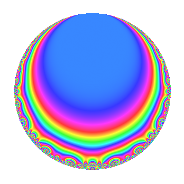# Properties

 Label 308.2.a.cLevel 308 Weight 2 Character orbit 308.a Self dual Yes Analytic conductor 2.459 Analytic rank 0 Dimension 3 CM No Inner twists 1

# Related objects

## Newspace parameters

 Level: $$N$$ = $$308 = 2^{2} \cdot 7 \cdot 11$$ Weight: $$k$$ = $$2$$ Character orbit: $$[\chi]$$ = 308.a (trivial)

## Newform invariants

 Self dual: Yes Analytic conductor: $$2.45939238226$$ Analytic rank: $$0$$ Dimension: $$3$$ Coefficient field: 3.3.1016.1 Coefficient ring: $$\Z[a_1, a_2, a_3]$$ Coefficient ring index: $$1$$ Fricke sign: $$-1$$ Sato-Tate group: $\mathrm{SU}(2)$

## $q$-expansion

Coefficients of the $$q$$-expansion are expressed in terms of a basis $$1,\beta_1,\beta_2$$ for the coefficient ring described below. We also show the integral $$q$$-expansion of the trace form.

 $$f(q)$$ $$=$$ $$q -\beta_{1} q^{3} + ( -\beta_{1} - \beta_{2} ) q^{5} + q^{7} + ( 1 + \beta_{1} + \beta_{2} ) q^{9} +O(q^{10})$$ $$q -\beta_{1} q^{3} + ( -\beta_{1} - \beta_{2} ) q^{5} + q^{7} + ( 1 + \beta_{1} + \beta_{2} ) q^{9} + q^{11} + ( 4 + \beta_{2} ) q^{13} + ( 2 + 3 \beta_{1} + \beta_{2} ) q^{15} + ( 2 \beta_{1} - \beta_{2} ) q^{17} -2 \beta_{1} q^{19} -\beta_{1} q^{21} + ( -2 - \beta_{1} + \beta_{2} ) q^{23} + ( 5 + 3 \beta_{1} - \beta_{2} ) q^{25} + ( -2 - \beta_{1} - \beta_{2} ) q^{27} + ( 2 - 2 \beta_{2} ) q^{29} + ( -4 + 3 \beta_{1} ) q^{31} -\beta_{1} q^{33} + ( -\beta_{1} - \beta_{2} ) q^{35} + ( 2 + \beta_{1} + \beta_{2} ) q^{37} + ( 2 - 6 \beta_{1} ) q^{39} + ( -2 \beta_{1} + \beta_{2} ) q^{41} + ( -2 + 2 \beta_{1} ) q^{43} + ( -10 - 4 \beta_{1} ) q^{45} + ( -2 + 2 \beta_{1} + \beta_{2} ) q^{47} + q^{49} + ( -10 - 2 \beta_{2} ) q^{51} + ( 2 \beta_{1} + 2 \beta_{2} ) q^{53} + ( -\beta_{1} - \beta_{2} ) q^{55} + ( 8 + 2 \beta_{1} + 2 \beta_{2} ) q^{57} + ( -8 + \beta_{1} ) q^{59} + ( 4 + 2 \beta_{1} + 3 \beta_{2} ) q^{61} + ( 1 + \beta_{1} + \beta_{2} ) q^{63} + ( -8 - 4 \beta_{1} - 2 \beta_{2} ) q^{65} + ( -2 + \beta_{1} - \beta_{2} ) q^{67} + ( 6 + \beta_{1} + \beta_{2} ) q^{69} + ( -2 + \beta_{1} + 3 \beta_{2} ) q^{71} + ( -4 - 2 \beta_{1} + \beta_{2} ) q^{73} + ( -14 - 6 \beta_{1} - 3 \beta_{2} ) q^{75} + q^{77} + ( 6 - 4 \beta_{1} - 2 \beta_{2} ) q^{79} + ( -1 + 2 \beta_{1} - 2 \beta_{2} ) q^{81} + ( 4 \beta_{1} - 2 \beta_{2} ) q^{83} + ( 4 - 6 \beta_{1} - 4 \beta_{2} ) q^{85} + ( -4 + 2 \beta_{1} ) q^{87} + ( -8 + 5 \beta_{1} + \beta_{2} ) q^{89} + ( 4 + \beta_{2} ) q^{91} + ( -12 + \beta_{1} - 3 \beta_{2} ) q^{93} + ( 4 + 6 \beta_{1} + 2 \beta_{2} ) q^{95} + ( 4 + 3 \beta_{1} + \beta_{2} ) q^{97} + ( 1 + \beta_{1} + \beta_{2} ) q^{99} +O(q^{100})$$ $$\operatorname{Tr}(f)(q)$$ $$=$$ $$3q - q^{3} - q^{5} + 3q^{7} + 4q^{9} + O(q^{10})$$ $$3q - q^{3} - q^{5} + 3q^{7} + 4q^{9} + 3q^{11} + 12q^{13} + 9q^{15} + 2q^{17} - 2q^{19} - q^{21} - 7q^{23} + 18q^{25} - 7q^{27} + 6q^{29} - 9q^{31} - q^{33} - q^{35} + 7q^{37} - 2q^{41} - 4q^{43} - 34q^{45} - 4q^{47} + 3q^{49} - 30q^{51} + 2q^{53} - q^{55} + 26q^{57} - 23q^{59} + 14q^{61} + 4q^{63} - 28q^{65} - 5q^{67} + 19q^{69} - 5q^{71} - 14q^{73} - 48q^{75} + 3q^{77} + 14q^{79} - q^{81} + 4q^{83} + 6q^{85} - 10q^{87} - 19q^{89} + 12q^{91} - 35q^{93} + 18q^{95} + 15q^{97} + 4q^{99} + O(q^{100})$$

Basis of coefficient ring in terms of a root $$\nu$$ of $$x^{3} - x^{2} - 6 x + 2$$:

 $$\beta_{0}$$ $$=$$ $$1$$ $$\beta_{1}$$ $$=$$ $$\nu$$ $$\beta_{2}$$ $$=$$ $$\nu^{2} - \nu - 4$$
 $$1$$ $$=$$ $$\beta_0$$ $$\nu$$ $$=$$ $$\beta_{1}$$ $$\nu^{2}$$ $$=$$ $$\beta_{2} + \beta_{1} + 4$$

## Embeddings

For each embedding $$\iota_m$$ of the coefficient field, the values $$\iota_m(a_n)$$ are shown below.

For more information on an embedded modular form you can click on its label.

Label $$\iota_m(\nu)$$ $$a_{2}$$ $$a_{3}$$ $$a_{4}$$ $$a_{5}$$ $$a_{6}$$ $$a_{7}$$ $$a_{8}$$ $$a_{9}$$ $$a_{10}$$
1.1
 2.85577 0.321637 −2.17741
0 −2.85577 0 −4.15544 0 1.00000 0 5.15544 0
1.2 0 −0.321637 0 3.89655 0 1.00000 0 −2.89655 0
1.3 0 2.17741 0 −0.741113 0 1.00000 0 1.74111 0
 $$n$$: e.g. 2-40 or 990-1000 Significant digits: Format: Complex embeddings Normalized embeddings Satake parameters Satake angles

## Inner twists

This newform does not admit any (nontrivial) inner twists.

## Atkin-Lehner signs

$$p$$ Sign
$$2$$ $$-1$$
$$7$$ $$-1$$
$$11$$ $$-1$$

## Hecke kernels

This newform can be constructed as the kernel of the linear operator $$T_{3}^{3} + T_{3}^{2} - 6 T_{3} - 2$$ acting on $$S_{2}^{\mathrm{new}}(\Gamma_0(308))$$.